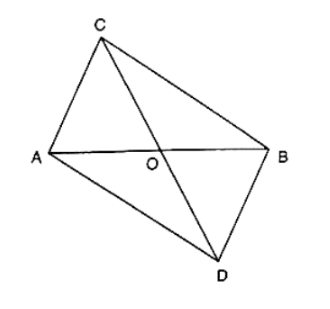# In figure, ABC and ABD are two triangles on the base AB.

Question:

In figure, ABC and ABD are two triangles on the base AB. If line segment CD is bisected by AB at O, show that ar(ΔABC) = ar(ΔABD).Solution:

Given that CD is bisected by AB at O

To prove: ar(ΔABC) = ar(ΔABD).

Construction: Draw CP ⊥ AB and DQ ⊥ AB.

Proof:

ar(ΔABC) = 1/2 × AB × CP⋅⋅⋅⋅⋅ (1)

ar(ΔABD) = 1/2 × AB × DQ ⋅⋅⋅⋅ (2)

In ΔCPO and ΔDQO

∠CPO = ∠DQO  [Each 90°]

Given that, CO = OD

∠COP = ∠DOQ  [Vertically opposite angles are equal]

Then, ΔCPO ≅ ΔDQO  [By AAS condition]

∴ CP = DQ (3)   [C.P.C.T]

Compare equation (1), (2) and (3)

∴ ar(ΔABC) = ar(ΔABD).